Courses

# Doc - MCQ:- Calculus, Integrals, Multiple, integrals Mathematics Notes | EduRev

## Topic-wise Tests & Solved Examples for IIT JAM Mathematics

Created by: Veda Institute

## Mathematics : Doc - MCQ:- Calculus, Integrals, Multiple, integrals Mathematics Notes | EduRev

The document Doc - MCQ:- Calculus, Integrals, Multiple, integrals Mathematics Notes | EduRev is a part of the Mathematics Course Topic-wise Tests & Solved Examples for IIT JAM Mathematics.
All you need of Mathematics at this link: Mathematics

Question:-1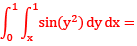Solution:
Region bounded  by
x = 0, x = 1 and y = x, y = 1
Now we evaluate the integral by hanging the order of integral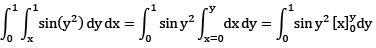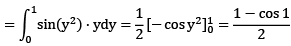Question:-2 The value of the double integral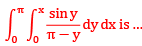Solution:
Region of integration is bounded  y=0, y = x and x = 0, x = π
changing the order of integration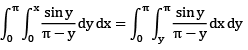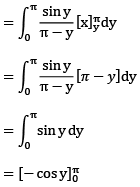= 2

Question:-3 Evaluate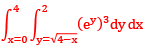Solution: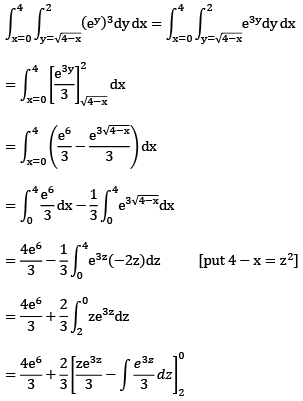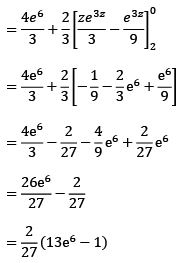Question4:- Change the order of integration in the double integral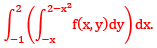Solution:
Domain of integration is bounded  by the following curves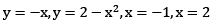point of intersection of the curve y =2 - x2 and y = -x we get when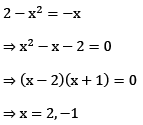So (-1,1 ) and (2,-2 ) are the points of intersection.
Now the given integral modify by changing the order of integration we get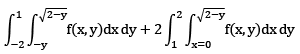Question5:- Changing the order of integration of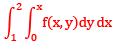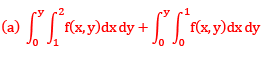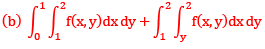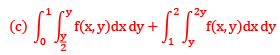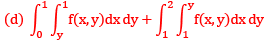Solution: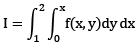The region of integration is bounded by y = 0, y = x, x = 1, x = 2 after changing the order the integration changes to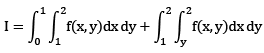Question6:- Let D the trianle bounded by the y-axis the line 2y = π. Then the value of the integral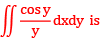(a) 1/2 (b) 1 (c) 3/2 (d) 2
Solution: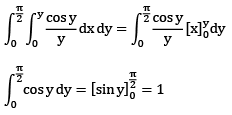Question7:- Change the order of integration in the integral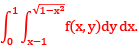Solution:  The domain of the double integration is bounded  by the curves y = x - 1,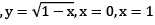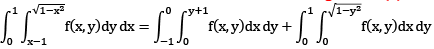Question8:- Let I =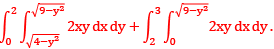Then using the transformation x = rcosθ, y = rsinθ, integral is equal to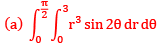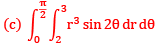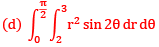Solution:
Region of integration is bounded  by curves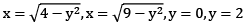for first integral,

Question9:- Evaluate integral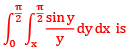(a) 0 (b) 1/2 (c) 1 (d)2
Solution:
Region of integration is bounded  by curves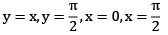changing the order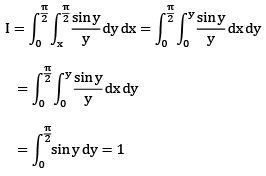Question 10:- The value of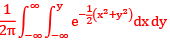equals
(a) π/4 (b) 1/2π (c) 1/4 (d) 1/2
Solution: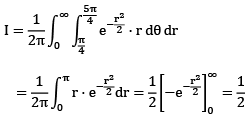Question11:- (a) Find in the area of the smaller of the two regions enclose between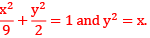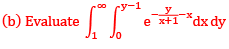Solution:-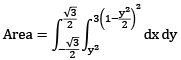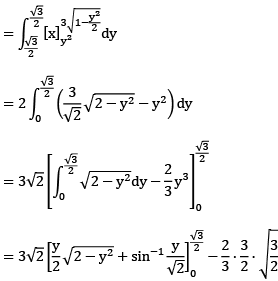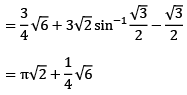Solution (b)
The region of integration is bounded  by x = 0, x = y, y = 1, y = ∞ and shown in figure changing the order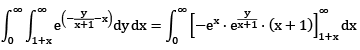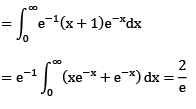Question12:- Let f ,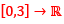be a continuous function with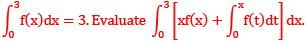Solution:
Given,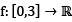be a continuous function with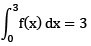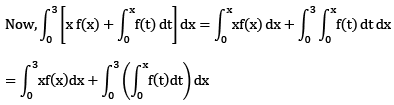Now apply change of order of integration
Domain of integration is given by graphQuestion13:- By changing the order of integration, the integral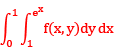can be expressed as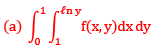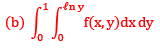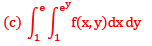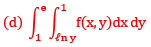Solution:
The domain of integration is bounded  y = 1,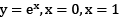changing the order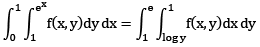Offer running on EduRev: Apply code STAYHOME200 to get INR 200 off on our premium plan EduRev Infinity!

,

,

,

,

,

,

,

,

,

,

,

,

,

,

,

,

,

,

,

,

,

,

,

,

,

,

,

,

,

,

;